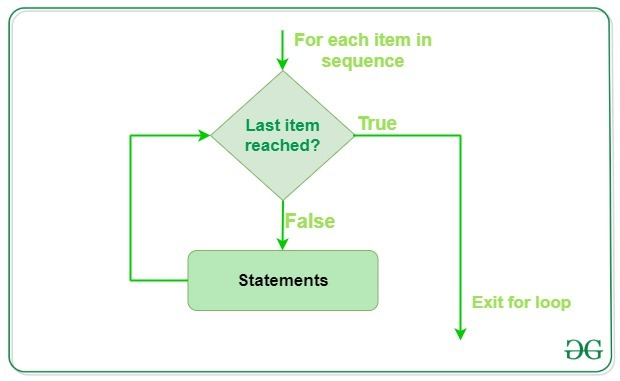# Python For Loops

• Difficulty Level : Easy
• Last Updated : 25 Aug, 2021

Python For loop is used for sequential traversal i.e. it is used for iterating over an iterable like string, tuple, list, etc. It falls under the category of definite iteration. Definite iterations mean the number of repetitions is specified explicitly in advance. In Python, there is no C style for loop, i.e., for (i=0; i<n; i++). There is “for in” loop which is similar to for each loop in other languages. Let us learn how to use for in loop for sequential traversals.

Note: In Python, for loops only implements the collection-based iteration.

Syntax:

```for var in iterable:
# statements```

### Flowchart of for loopHere the iterable is a collection of objects like lists, tuples. The indented statements inside the for loops are executed once for each item in an iterable. The variable var takes the value of the next item of the iterable each time through the loop.

## Python3

 `# Python program to illustrate` `# Iterating over a list` `print``(``"List Iteration"``)` `l ``=` `[``"geeks"``, ``"for"``, ``"geeks"``]` `for` `i ``in` `l:` `    ``print``(i)`   `# Iterating over a tuple (immutable)` `print``(``"\nTuple Iteration"``)` `t ``=` `(``"geeks"``, ``"for"``, ``"geeks"``)` `for` `i ``in` `t:` `    ``print``(i)`   `# Iterating over a String` `print``(``"\nString Iteration"``)` `s ``=` `"Geeks"` `for` `i ``in` `s:` `    ``print``(i)`   `# Iterating over dictionary` `print``(``"\nDictionary Iteration"``)` `d ``=` `dict``()` `d[``'xyz'``] ``=` `123` `d[``'abc'``] ``=` `345` `for` `i ``in` `d:` `    ``print``(``"% s % d"` `%` `(i, d[i]))`

Output:

```List Iteration
geeks
for
geeks

Tuple Iteration
geeks
for
geeks

String Iteration
G
e
e
k
s

Dictionary Iteration
xyz 123
abc 345```

## Loop Control Statements

Loop control statements change execution from its normal sequence. When execution leaves a scope, all automatic objects that were created in that scope are destroyed. Python supports the following control statements.

### Continue Statement

Python continue Statement returns the control to the beginning of the loop.

## Python3

 `# Prints all letters except 'e' and 's'` `for` `letter ``in` `'geeksforgeeks'``:` `    ``if` `letter ``=``=` `'e'` `or` `letter ``=``=` `'s'``:` `        ``continue` `    ``print``(``'Current Letter :'``, letter)`

Output:

```Current Letter : g
Current Letter : k
Current Letter : f
Current Letter : o
Current Letter : r
Current Letter : g
Current Letter : k```

### Break Statement

Python break statement brings control out of the loop.

## Python3

 `for` `letter ``in` `'geeksforgeeks'``:`   `    ``# break the loop as soon it sees 'e'` `    ``# or 's'` `    ``if` `letter ``=``=` `'e'` `or` `letter ``=``=` `'s'``:` `        ``break`   `print``(``'Current Letter :'``, letter)`

Output:

`Current Letter : e`

### Pass Statement

The pass statement to write empty loops. Pass is also used for empty control statements, functions, and classes.

## Python3

 `# An empty loop` `for` `letter ``in` `'geeksforgeeks'``:` `    ``pass` `print``(``'Last Letter :'``, letter)`

Output:

`Last Letter : s `

## range() function

Python range() is a built-in function that is used when a user needs to perform an action a specific number of times. range() in Python(3.x) is just a renamed version of a function called xrange() in Python(2.x). The range() function is used to generate a sequence of numbers. Depending on how many arguments user is passing to the function, user can decide where that series of numbers will begin and end as well as how big the difference will be between one number and the next.range() takes mainly three arguments.

• start: integer starting from which the sequence of integers is to be returned
• stop: integer before which the sequence of integers is to be returned.
The range of integers end at stop – 1.
• step: integer value which determines the increment between each integer in the sequence

## Python3

 `# Python Program to` `# show range() basics`   `# printing a number` `for` `i ``in` `range``(``10``):` `    ``print``(i, end``=``" "``)` `print``()`   `# using range for iteration` `l ``=` `[``10``, ``20``, ``30``, ``40``]` `for` `i ``in` `range``(``len``(l)):` `    ``print``(l[i], end``=``" "``)` `print``()`   `# performing sum of first 10 numbers` `sum` `=` `0` `for` `i ``in` `range``(``1``, ``10``):` `    ``sum` `=` `sum` `+` `i` `print``(``"Sum of first 10 numbers :"``, ``sum``)`

Output

```0 1 2 3 4 5 6 7 8 9
10 20 30 40
Sum of first 10 numbers : 45```

## Python for loop with else

In most of the programming languages (C/C++, Java, etc), the use of else statements has been restricted with the if conditional statements. But Python also allows us to use the else condition with for loops.

Note: The else block just after for/while is executed only when the loop is NOT terminated by a break statement

## Python3

 `# Python program to demonstrate` `# for-else loop`   `for` `i ``in` `range``(``1``, ``4``):` `    ``print``(i)` `else``:  ``# Executed because no break in for` `    ``print``(``"No Break\n"``)`   `for` `i ``in` `range``(``1``, ``4``):` `    ``print``(i)` `    ``break` `else``:  ``# Not executed as there is a break` `    ``print``(``"No Break"``)`

Output:

```1
2
3
No Break

1```

Note: For more information refer to our Python for loop with else tutorial.

My Personal Notes arrow_drop_up